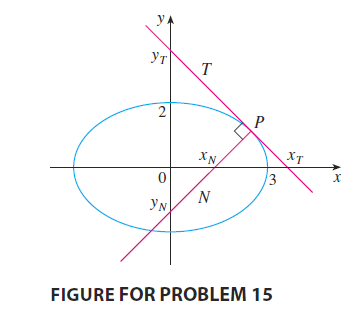#### Problem 15P

15. Let $T$ and $N$ be the tangent and normal lines to the ellipse $x^{2} / 9+y^{2} / 4=1$ at any point $P$ on the ellipse in the first quadrant. Let $x_{T}$ and $y_{T}$ be the $x$ – and $y$ -intercepts of $T$ and $x_{N}$ and $y_{N}$ be the intercepts of $N$. As $P$ moves along the ellipse in the first quadrant (but not on the axes), what values can $x_{T}, y_{T}, x_{N}$, and $y_{N}$ take on? First try to guess the answers just by looking at the figure. Then use calculus to solve the problem and see how good your intuition is.